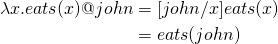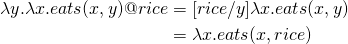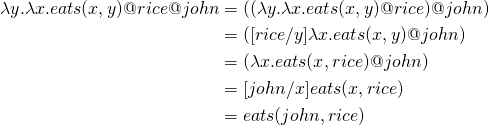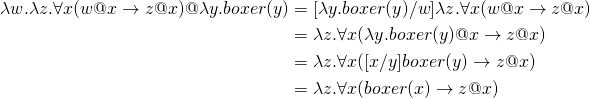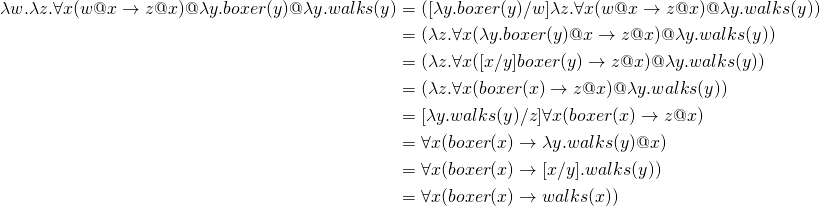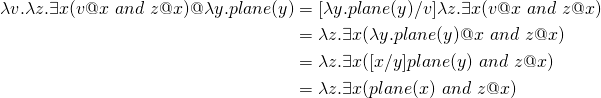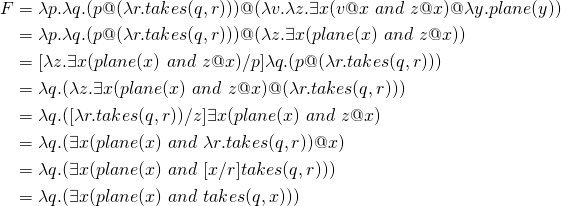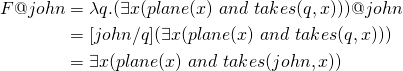# Primer on Lambda Calculus

## Expression

The fundamental concept in lambda calculus is the notion if an expression. An expression is defined as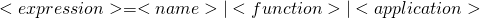## Name

A name, also called a variable, is an identifier which, in general, can be any of the letters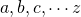. For example,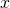.

## Function

An function is defined as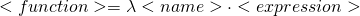An example of a function is the following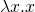This expression defines the identity function. The name after the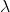is the identifier or the argument of this function. The expression after the point (in this case a single) is called the body of the function.

Functions can be applied to expressions.

## Application

An application is defined as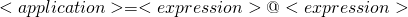An example of an application is the following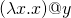This is the identity function applied to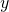. Parenthesis are used for clarity in order to avoid ambiguity. Function applications are evaluated by substituting the value of the argument(in this case) in the body of the function definition, i.e.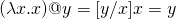In this transformation the notation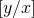is used to indicate that all occurrences ofare substituted byin the expression to the right.

## Order of Evaluation

An expression can be surrounded with parenthesis for clarity, that is, if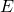is an expression,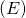is the same expression. The only keywords used in the language areand the dot. In order to avoid cluttering expressions with parenthesis, the convention is that function application associates from the left.

For example the expression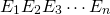is evaluated applying the expressions as follows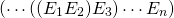## Scope

Incalculus all names are local to definitions. In the function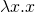,is bound since its occurrence in the body of the definition is preceded by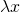. A name not preceded by ais called a free variable. In the expression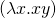the variableis bound andis free. In the expression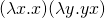Thein the body of the first expression from the left is bound to the first. Thein the body of the second expression is bound to the secondand theis free. It is very important to notice that thein the second expression is totally independent of thein the first expression.

Be careful when performing substitutions to avoid mixing up free occurrences of an identifier with bound ones. In the expression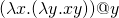the function to the left contains a bound, whereas theat the right is free. Simply by renaming the boundto, this confusion is avoided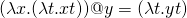## Examples